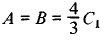# Exhaustion, Method of

The following article is from The Great Soviet Encyclopedia (1979). It might be outdated or ideologically biased.

## Exhaustion, Method of

a method of proof used by the mathematicians of antiquity to find areas and volumes. The term “method of exhaustion” was introduced in the 17th century.

A typical scheme of proof using the method of exhaustion may be stated in modern terminology as follows. In order to determine the quantity A, one constructs a certain sequence of quantities C1, Ci, …, Cn such that

(1) Cn < A

One also supposes that a B is known such that

(2) Cn < B

and for any integer K the inequalities

(3) K(A - Cn)< DK(B - Cn)< D

where D is a constant, are satisfied for sufficiently large n. From the modern viewpoint, in order to proceed from the inequalities (3) to the equality

(4) A = B

it is sufficient to note that from the conditions (1), (2), and (3) there follows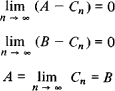The mathematicians of antiquity, who did not have the theory of limits available, turned to proof by contradiction and showed the impossibility of each of the inequalities A < B, B < A. In order to refute the first of these, they established, with the help of the Eudoxus-Archimedes axiom, that for R = BA there exists a K such that KR > D, and on the strength of condition (1) they obtained

K (BCn) > K (BA) > D

which contradicts the second of the inequalities (3). The other assumption was similarly refuted. After this, only equality (4) remained to be accepted.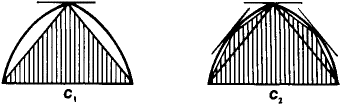Figure 1

The introduction of the method of exhaustion together with the axiom on which it is based is attributed to Eudoxus of Cnidos. This method was widely used by Euclid and with exceptional skill and variety by Archimedes. For example, in order to determine the area of a parabolic segment A, Archimedes constructed the areas C1, C2, …, which “exhaust” by their gradual growth the area A of the segment according to the scheme that is evident from Figure 1. In this case,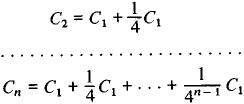Instead of resorting to passage to the limit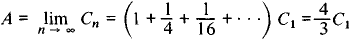Archimedes geometrically proved that for any n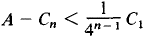Introducing the area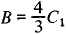Archimedes obtained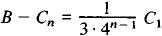and, following the procedure presented above, completed the proof that